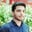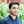Trusted answers to developer questions
Trusted Answers to Developer Questions

Related Tags

python
communitycreator

# What is math.atan2() in Python?Salman Yousaf

Grokking Modern System Design Interview for Engineers & Managers

Ace your System Design Interview and take your career to the next level. Learn to handle the design of applications like Netflix, Quora, Facebook, Uber, and many more in a 45-min interview. Learn the RESHADED framework for architecting web-scale applications by determining requirements, constraints, and assumptions before diving into a step-by-step design process.

### Overview

This math.atan2() method is used to calculate the arctangent of y/x, in radians, where x and y are coordinates of a point (x,y) in the cartesian plane.

The value returned by this function is between -π and π.

atan2(y,x)

### Syntax

math.atan2(y, x)


### Parameters

This method accepts positive and negative numeric values.

• y: the first parameter
• x: the second parameter

### Return value

This method returns a primitive float value, representing the arc tangent x/y in radians, between -π and π.

This angle θ returned by this method is from polar coordinate (r,θ).

### Code

import math

# Two positive coordinates
print(math.atan2(3, 4))

# Two negative coordinates
print(math.atan2(-3, -4))

# One positive and One negative coordinate
print(math.atan2(3.4, -3.1))

### TypeError

This method will generate an error when we pass integer arguments.

It’s also Python version-dependent.

• Python 3: TypeError: a float is required.

• Python 3.8: TypeError: must be a real number, not a list.

#### Example 1: Python 3 TypeError

import math# It will generate type error on integer argumenty, x = 2, 3theeta = math.atan2([y], [x])print(theeta)
Python 3

#### Example 2: Python 3.8 TypeError

import math# It will generate type error on integer argumenty, x = 2, 3theeta = math.atan2([y], [x])print(theeta)
Python 3.8

RELATED TAGS

python
communitycreator

CONTRIBUTORSalman Yousaf

Grokking Modern System Design Interview for Engineers & Managers

Ace your System Design Interview and take your career to the next level. Learn to handle the design of applications like Netflix, Quora, Facebook, Uber, and many more in a 45-min interview. Learn the RESHADED framework for architecting web-scale applications by determining requirements, constraints, and assumptions before diving into a step-by-step design process.

Keep Exploring

Learn in-demand tech skills in half the time